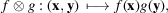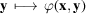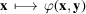International
Tables for
Crystallography
Volume B
Reciprocal space
Edited by U. Shmueli

International Tables for Crystallography (2010). Vol. B, ch. 1.3, pp. 33-34   | 1 | 2 |

## Section 1.3.2.3.9.6. Tensor product of distributions

G. Bricognea

aGlobal Phasing Ltd, Sheraton House, Suites 14–16, Castle Park, Cambridge CB3 0AX, England, and LURE, Bâtiment 209D, Université Paris-Sud, 91405 Orsay, France

#### 1.3.2.3.9.6. Tensor product of distributions

| top | pdf |

The purpose of this construction is to extend Fubini's theorem to distributions. Following Section 1.3.2.2.5, we may define the tensor productas the vector space of finite linear combinations of functions of the formwhereand.

Letanddenote the distributions associated to f and g, respectively, the subscripts x and y acting as mnemonics forand. It follows from Fubini's theorem (Section 1.3.2.2.5) that, and hence defines a distribution over; the rearrangement of integral signs givesfor all. In particular, ifwith, thenThis construction can be extended to general distributionsand. Given any test function, letdenote the map; letdenote the map; and define the two functionsand. Then, by the lemma on differentiation under thesign of Section 1.3.2.3.9.1,, and there exists a unique distributionsuch thatis called the tensor product of S and T.

With the mnemonic introduced above, this definition reads identically to that given above for distributions associated to locally integrable functions:The tensor product of distributions is associative:Derivatives may be calculated byThe support of a tensor product is the Cartesian product of the supports of the two factors.